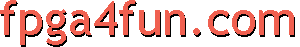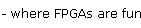Opto 2 - Varying an LED intensity

Turning an LED on and off

Here's how to make an LED blink (on and off).

input clk;     // clock typically from 10MHz to 50MHz
output LED;

// create a binary counter
reg [31:0] cnt;
always @(posedge clk) cnt <= cnt+1;

assign LED = cnt;    // blink the LED at a few Hz (using the 23th bit of the counter, use a different bit to modify the blinking rate)
endmodule
Making an LED half-lit

One solution would be doubling the value of the resistor used in series with the LED. Another solution is driving the LED FPGA output half of the time "fast enough", so that your eye average the light and see the LED half-lit.

module LEDhalflit(clk, LED);
input clk;
output LED;

reg toggle;
always @(posedge clk) toggle <= ~toggle;     // toggles at half the clk frequency

assign LED = toggle;
endmodule
Fine-tuning the LED intensity

For more LED intensity control, a PWM is an ideal solution.
Here's an example that uses a 4-bits control to select between 16 intensity levels out of an LED.

module LED_PWM(clk, PWM_input, LED);
input clk;
input [3:0] PWM_input;     // 16 intensity levels
output LED;

reg [4:0] PWM;
always @(posedge clk) PWM <= PWM[3:0] + PWM_input;

assign LED = PWM;
endmodule

By continuously changing the LED intensity, the LED appears to "glow".

module LEDglow(clk, LED);
input clk;
output LED;

reg [23:0] cnt;
always @(posedge clk) cnt <= cnt+1;

reg [4:0] PWM;
wire [3:0] intensity = cnt ? cnt[22:19] : ~cnt[22:19];    // ramp the intensity up and down
always @(posedge clk) PWM <= PWM[3:0] + intensity;

assign LED = PWM;
endmodule

This is the same example that was presented in part 0.